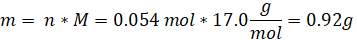# Chemistry

Some of the content of this guide was modeled after a guide originally created by Openstax and has been adapted for the GPRC Learning Commons in April 2021.Limiting Reagent

The limiting reagent in a chemical reaction is the substance that gets completely consumed first.  An introduction to limiting reagents is given in the Stoichiometry section. This section includes an example with the steps that will help you find the limiting reagent of a chemical reaction.

Example 1

In the following reaction, 2.25 g of NH3 is allowed to react with 2.50 g of O2. With that information, answer the following questions:

NH3 + O2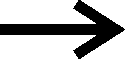NO + H2O

1. In the above reaction, what is the limiting reagent?
2. How many grams of NO are formed?
3. How much of the excess reactant remains after the completion of the reaction?

Step 1

Find the mols of O2 and NH3 using the following formula: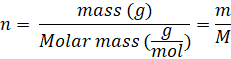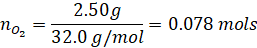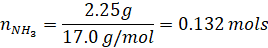Finding the limiting reagent:

 Substance Coefficient from the balanced equation Number of mols available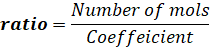Limiting reagent O2 1 0.078 mols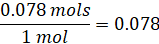The one with the smallest ratio becomes the limiting reagent. In this case, it’s O2 with the smallest ratio of 0.078 NH3 1 0.132 mols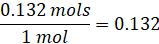Step 2

Finding the amount of NO produced using the number of mols of O2 since it’s the limiting reagent and it’s going to dictate how much NO can be produced.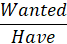NO O2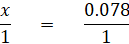“Have” comes from the coefficient of balanced chemical equation

Solving for x in the above equation gives 0.078 mols for x. Therefore, the amount of NO produced is 0.078 mols - with that, we can calculate the amount of NO produced in grams using the following formula:

Molar mass of NO = 30.01g/mol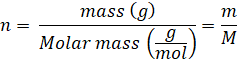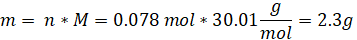Step 3

Finding the amount of NH3 that’s left. In order to find the amount of NH3 left, we first have to find the amount of NH3 used. It can be found using the molar ratios, as shown below:NH3 O2“Have” comes from the coefficient of balanced chemical equation

Solving for x in the above equation gives 0.078 mols for x. Therefore, the amount of NH3 used is 0.078 mols, and the amount of NH3 left is found as follows:

NH3 left = 0.132 mols – 0.078 mols = 0.054 mols

The mass of NH3 left can be found using the following equation: